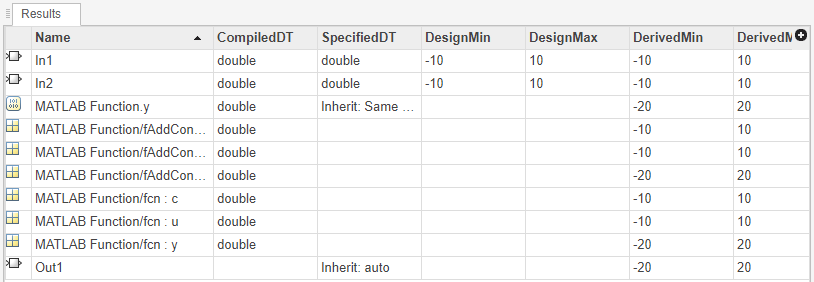# Troubleshoot Range Analysis of System Objects

When deriving ranges for a model that uses a System object™, the analysis fails if the model contains variables that can refer to multiple handle objects. The following example shows how to reconfigure the code so that the Fixed-Point Tool can derive ranges for the model.

In this example, range analysis of the first model `ex_HandleVariableRefersToMultipleObjects` produces an error because there is a variable in the code that can refer to different system objects depending on other conditions. The model `ex_HandleVariableRefersToSingleObject` is a rewrite of the first model with the same functionality, but the Fixed-Point Tool is able to derive ranges for the model.

### Derive Ranges for Model with System Object

Open the first model, `ex_HandleVariableRefersToMultipleObjects`.

`open_system("ex_HandleVariableRefersToMultipleObjects.slx")`

The code inside the MATLAB Function block refers to the custom System object `fAddConstant`.

```function y = fcn(u, c) %#codegen persistent hSysObjAddTen persistent hSysObjAddNegTen persistent hSysObjForStep if isempty(hSysObjAddTen) hSysObjAddTen = fAddConstant(10); end if isempty(hSysObjAddNegTen) hSysObjAddNegTen = fAddConstant(-10); end if c > 0 hSysObjForStep = hSysObjAddTen; else hSysObjForStep = hSysObjAddNegTen; end y = step(hSysObjForStep, u); ```

From the Simulink® Apps tab, select Fixed-Point Tool.

In the Fixed-Point Tool, select the Iterative Fixed-Point Conversion workflow.

Under System Under Design (SUD), select `ex_HandleVariableRefersToMultipleObjects` as the system you want to convert.

Under Range Collection Mode, select Derived ranges.

Click the Collect Ranges button. The analysis fails because there is a handle variable in the code that can refer to different system objects depending on the value of `c`.

### Rewrite Code to Enable Derived Range Analysis

You can rewrite the code inside the MATLAB Function block so that the Fixed-Point Tool is able to derive ranges for the System object.

Close the Fixed-Point Tool and the `ex_HandleVariableRefersToMultipleObjects` model. Open the `ex_HandleVariableRefersToSingleObject` model.

`open_system("ex_HandleVariableRefersToSingleObject.slx")`

This model contains the rewritten code:

```function y = fcn(u, c) %#codegen persistent hSysObjAddTen persistent hSysObjAddNegTen if isempty(hSysObjAddTen) hSysObjAddTen = fAddConstant(10); end if isempty(hSysObjAddNegTen) hSysObjAddNegTen = fAddConstant(-10); end if c > 0 y = step(hSysObjAddTen, u); else y = step(hSysObjAddNegTen, u); end ```

From the Simulink Apps tab, select Fixed-Point Tool.

In the Fixed-Point Tool, select the Iterative Fixed-Point Conversion workflow.

Under System Under Design (SUD), select `ex_HandleVariableRefersToSingleObject` as the system you want to convert.

Under Range Collection Mode, select Derived ranges.

Click the Collect Ranges button. This time, the Fixed-Point Tool successfully derives ranges for the variables used in the model.